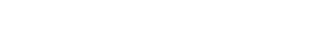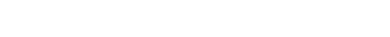# Bivariate Normal Distribution / Multivariate Normal (Overview)

Contents:

1. Bivariate Normal
2. Multivariate Normal

## 1. What is a Bivariate Normal Distribution?

The “regular” normal distribution has one random variable; A bivariate normal distribution is made up of two independent random variables. The two variables in a bivariate normal are both are normally distributed, and they have a normal distribution when both are added together. Visually, the bivariate normal distribution is a three-dimensional bell curve.

Francis Galton (1822-1911) was one of the first mathematicians to study the bivariate normal distribution in depth, during his study on the heights of parents and their adult children. Bravais, Gauss, Laplace, Plana also studied the distribution in the early nineteenth century (Balakrishnan & Lai, 2009).

The bivariare distribution can be described in many different ways and as such, there isn’t a unified agreement for a succinct definition. Some of the more common ways to characterize it include:

• Random variables X & Y are bivariate normal if aX + bY has a normal distribution for all a,b∈R.
• X and Y are jointly normal if they can be expressed as X = aU + bV, and Y = cU + dV (Bertsekas & Tsitsiklis, 2002)
• If a and b are non-zero constants, aX + bY has a normal distribution (Johnson & Kotz, 1972).
• If X – aY and Y are independent and if Y – bx and X are independent for all a,b (such that ab ≠ 0 or 1), then (X,Y) has a normal distribution (Rao, 1975).

There are literally dozens of different variants of these definitions. That’s one reason why the bivariate normal is usually defined in terms of its PDF.

## PDF of the Bivariate Normal Distribution.

The bivariate normal distribution can be defined as the probability density function (PDF) of two variables X and Y that are linear functions of the same independent normal random variables (adapted from Wolfram):Where:

If p = 2, this is equal to the bivariate normal distribution.

For some excellent gifs that show what happens when a few of these parameters are changed, check out Brad Hartlaub’s page at Kenyon college. This one shows what happens when μ1 is changed:## 2. What is a Multivariate Normal Distribution?

The multivariate normal distribution has two or more random variables — so the bivariate normal distribution is actually a special case of the multivariate normal distribution. That said, while the bivariate normal can be easily visualized (as demonstrated in the gif above), more than two variables poses problems with visualization. Thus, the multinormal can be difficult to wrap your head around — at least, visually. That said, if you’re familiar with matrix algebra it’s fairly easy to work with, and is one of the most important distributions in multivariate statistics.

The multivariate normal distribution is most often described by its joint density function. A multivariate normal p x 1 random vector X, with population mean vector μ and population variance-covariance matrix σ, will have the following joint density function:Where:

• |Σ| = determinant of the variance-covariance matrix Σ
• Σ-1 = inverse of the variance-covariance matrix Σ

References:
Balakrishnan,N. & Lai, C. (2009) Continuous Bivariate Distributions.
Johnson & Kotz. (1972) Distributions in Statistics: Continuous Multivariate Distributions.
Bertsekas & Tsitsiklis (2002). Introduction to Probability (1st ed.).
Rao, C. (1975). Some Problems in the Characterization of the Multivariate Normal Distribution.
Wolfram Mathworld. BND. Retrieved August 4, 2017 from: http://mathworld.wolfram.com/BivariateNormalDistribution.html

CITE THIS AS:
Stephanie Glen. "Bivariate Normal Distribution / Multivariate Normal (Overview)" From StatisticsHowTo.com: Elementary Statistics for the rest of us! https://www.statisticshowto.com/bivariate-normal-distribution/
---------------------------------------------------------------------------Need help with a homework or test question? With Chegg Study, you can get step-by-step solutions to your questions from an expert in the field. Your first 30 minutes with a Chegg tutor is free!